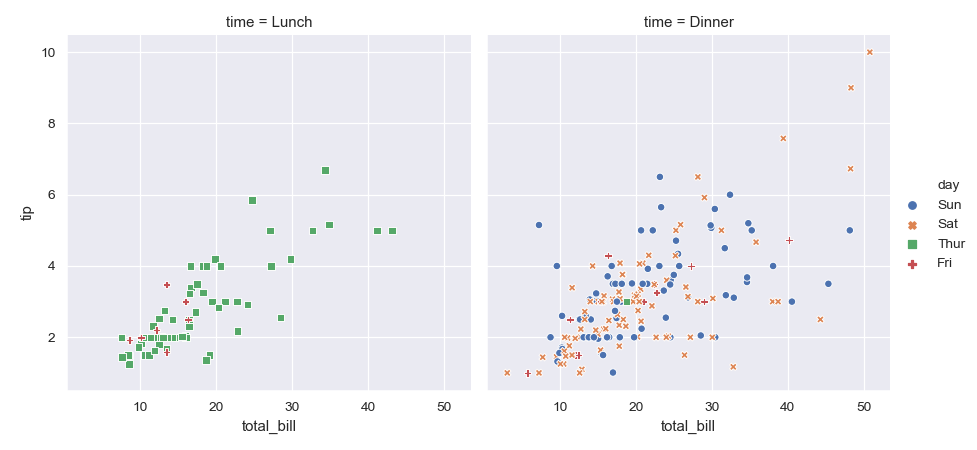# seaborn.scatterplot¶

`seaborn.``scatterplot`(x=None, y=None, hue=None, style=None, size=None, data=None, palette=None, hue_order=None, hue_norm=None, sizes=None, size_order=None, size_norm=None, markers=True, style_order=None, x_bins=None, y_bins=None, units=None, estimator=None, ci=95, n_boot=1000, alpha='auto', x_jitter=None, y_jitter=None, legend='brief', ax=None, **kwargs)

Draw a scatter plot with possibility of several semantic groupings.

The relationship between `x` and `y` can be shown for different subsets of the data using the `hue`, `size`, and `style` parameters. These parameters control what visual semantics are used to identify the different subsets. It is possible to show up to three dimensions independently by using all three semantic types, but this style of plot can be hard to interpret and is often ineffective. Using redundant semantics (i.e. both `hue` and `style` for the same variable) can be helpful for making graphics more accessible.

The default treatment of the `hue` (and to a lesser extent, `size`) semantic, if present, depends on whether the variable is inferred to represent “numeric” or “categorical” data. In particular, numeric variables are represented with a sequential colormap by default, and the legend entries show regular “ticks” with values that may or may not exist in the data. This behavior can be controlled through various parameters, as described and illustrated below.

Parameters
x, ynames of variables in `data` or vector data, optional

Input data variables; must be numeric. Can pass data directly or reference columns in `data`.

huename of variables in `data` or vector data, optional

Grouping variable that will produce points with different colors. Can be either categorical or numeric, although color mapping will behave differently in latter case.

sizename of variables in `data` or vector data, optional

Grouping variable that will produce points with different sizes. Can be either categorical or numeric, although size mapping will behave differently in latter case.

stylename of variables in `data` or vector data, optional

Grouping variable that will produce points with different markers. Can have a numeric dtype but will always be treated as categorical.

Tidy (“long-form”) dataframe where each column is a variable and each row is an observation.

palettepalette name, list, or dict, optional

Colors to use for the different levels of the `hue` variable. Should be something that can be interpreted by `color_palette()`, or a dictionary mapping hue levels to matplotlib colors.

hue_orderlist, optional

Specified order for the appearance of the `hue` variable levels, otherwise they are determined from the data. Not relevant when the `hue` variable is numeric.

hue_normtuple or Normalize object, optional

Normalization in data units for colormap applied to the `hue` variable when it is numeric. Not relevant if it is categorical.

sizeslist, dict, or tuple, optional

An object that determines how sizes are chosen when `size` is used. It can always be a list of size values or a dict mapping levels of the `size` variable to sizes. When `size` is numeric, it can also be a tuple specifying the minimum and maximum size to use such that other values are normalized within this range.

size_orderlist, optional

Specified order for appearance of the `size` variable levels, otherwise they are determined from the data. Not relevant when the `size` variable is numeric.

size_normtuple or Normalize object, optional

Normalization in data units for scaling plot objects when the `size` variable is numeric.

markersboolean, list, or dictionary, optional

Object determining how to draw the markers for different levels of the `style` variable. Setting to `True` will use default markers, or you can pass a list of markers or a dictionary mapping levels of the `style` variable to markers. Setting to `False` will draw marker-less lines. Markers are specified as in matplotlib.

style_orderlist, optional

Specified order for appearance of the `style` variable levels otherwise they are determined from the data. Not relevant when the `style` variable is numeric.

{x,y}_binslists or arrays or functions

Currently non-functional.

units{long_form_var}

Grouping variable identifying sampling units. When used, a separate line will be drawn for each unit with appropriate semantics, but no legend entry will be added. Useful for showing distribution of experimental replicates when exact identities are not needed.

Currently non-functional.

estimatorname of pandas method or callable or None, optional

Method for aggregating across multiple observations of the `y` variable at the same `x` level. If `None`, all observations will be drawn. Currently non-functional.

ciint or “sd” or None, optional

Size of the confidence interval to draw when aggregating with an estimator. “sd” means to draw the standard deviation of the data. Setting to `None` will skip bootstrapping. Currently non-functional.

n_bootint, optional

Number of bootstraps to use for computing the confidence interval. Currently non-functional.

alphafloat

Proportional opacity of the points.

{x,y}_jitterbooleans or floats

Currently non-functional.

legend“brief”, “full”, or False, optional

How to draw the legend. If “brief”, numeric `hue` and `size` variables will be represented with a sample of evenly spaced values. If “full”, every group will get an entry in the legend. If `False`, no legend data is added and no legend is drawn.

axmatplotlib Axes, optional

Axes object to draw the plot onto, otherwise uses the current Axes.

kwargskey, value mappings

Other keyword arguments are passed down to `matplotlib.axes.Axes.scatter()`.

Returns
axmatplotlib Axes

Returns the Axes object with the plot drawn onto it.

`lineplot`

Show the relationship between two variables connected with lines to emphasize continuity.

`swarmplot`

Draw a scatter plot with one categorical variable, arranging the points to show the distribution of values.

Examples

Draw a simple scatter plot between two variables:

```>>> import seaborn as sns; sns.set()
>>> import matplotlib.pyplot as plt
>>> ax = sns.scatterplot(x="total_bill", y="tip", data=tips)
```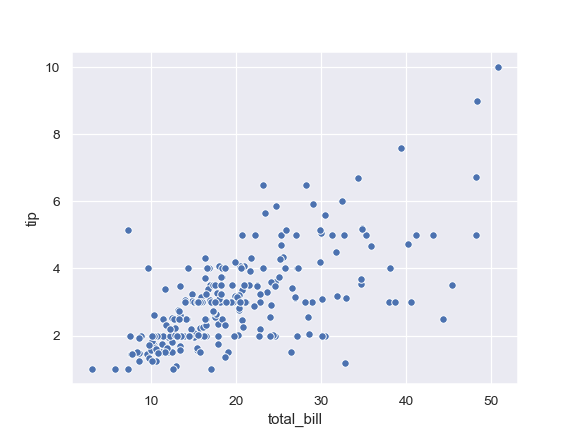Group by another variable and show the groups with different colors:

```>>> ax = sns.scatterplot(x="total_bill", y="tip", hue="time",
...                      data=tips)
```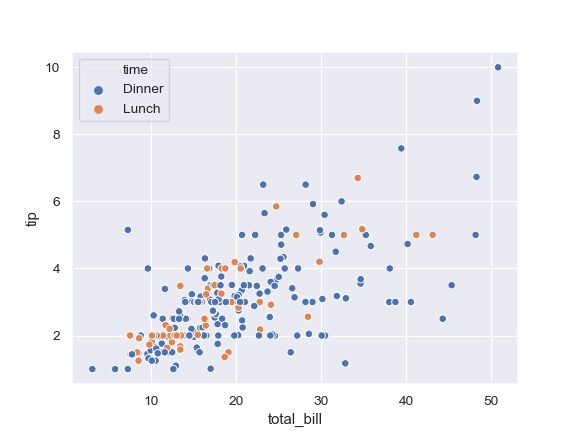Show the grouping variable by varying both color and marker:

```>>> ax = sns.scatterplot(x="total_bill", y="tip",
...                      hue="time", style="time", data=tips)
```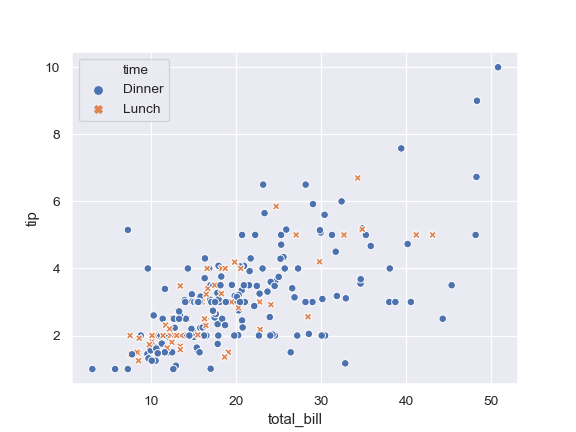Vary colors and markers to show two different grouping variables:

```>>> ax = sns.scatterplot(x="total_bill", y="tip",
...                      hue="day", style="time", data=tips)
```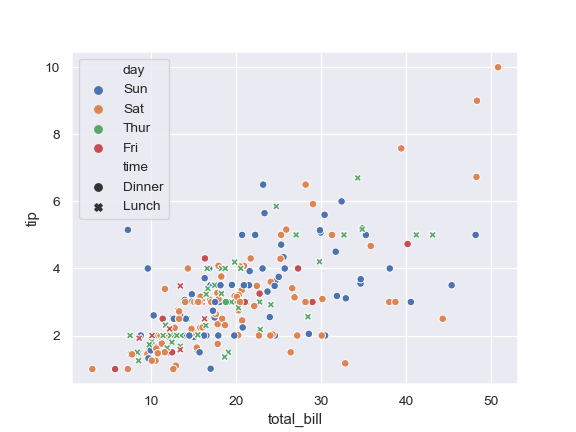Show a quantitative variable by varying the size of the points:

```>>> ax = sns.scatterplot(x="total_bill", y="tip", size="size",
...                      data=tips)
```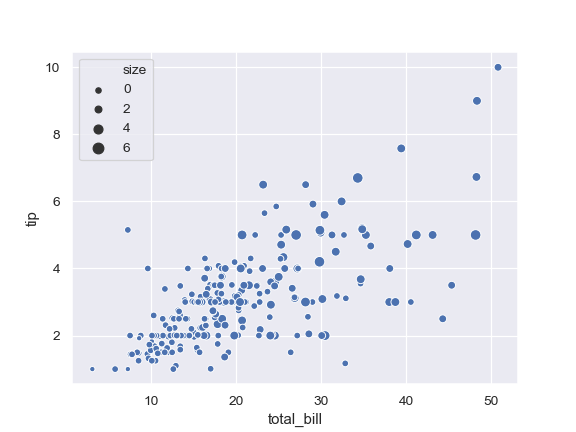Also show the quantitative variable by also using continuous colors:

```>>> ax = sns.scatterplot(x="total_bill", y="tip",
...                      hue="size", size="size",
...                      data=tips)
```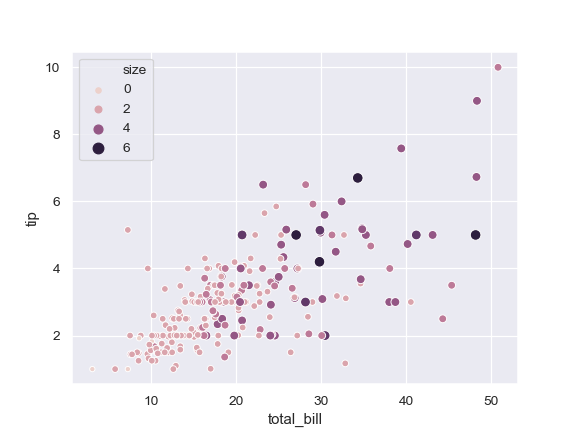Use a different continuous color map:

```>>> cmap = sns.cubehelix_palette(dark=.3, light=.8, as_cmap=True)
>>> ax = sns.scatterplot(x="total_bill", y="tip",
...                      hue="size", size="size",
...                      palette=cmap,
...                      data=tips)
```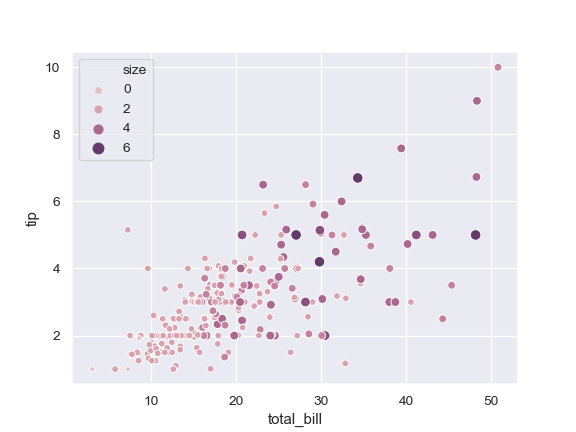Change the minimum and maximum point size and show all sizes in legend:

```>>> cmap = sns.cubehelix_palette(dark=.3, light=.8, as_cmap=True)
>>> ax = sns.scatterplot(x="total_bill", y="tip",
...                      hue="size", size="size",
...                      sizes=(20, 200), palette=cmap,
...                      legend="full", data=tips)
```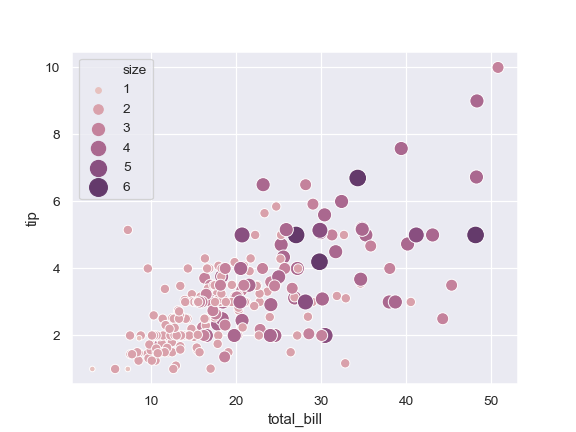Use a narrower range of color map intensities:

```>>> cmap = sns.cubehelix_palette(dark=.3, light=.8, as_cmap=True)
>>> ax = sns.scatterplot(x="total_bill", y="tip",
...                      hue="size", size="size",
...                      sizes=(20, 200), hue_norm=(0, 7),
...                      legend="full", data=tips)
```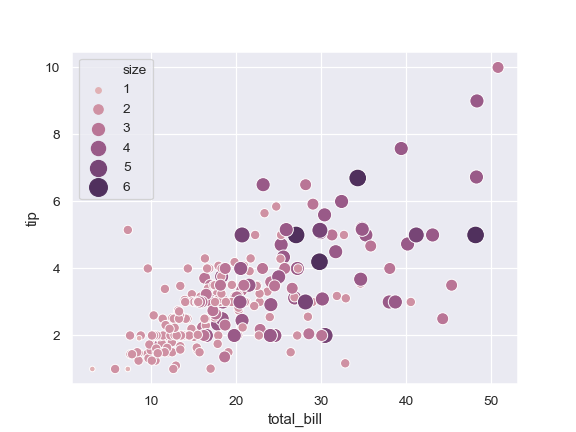Vary the size with a categorical variable, and use a different palette:

```>>> cmap = sns.cubehelix_palette(dark=.3, light=.8, as_cmap=True)
>>> ax = sns.scatterplot(x="total_bill", y="tip",
...                      hue="day", size="smoker",
...                      palette="Set2",
...                      data=tips)
```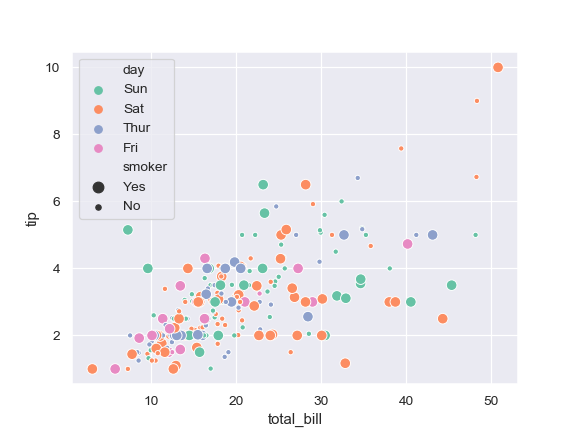Use a specific set of markers:

```>>> markers = {"Lunch": "s", "Dinner": "X"}
>>> ax = sns.scatterplot(x="total_bill", y="tip", style="time",
...                      markers=markers,
...                      data=tips)
```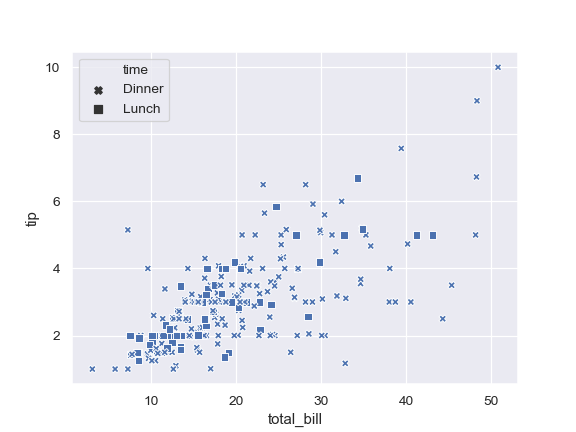Control plot attributes using matplotlib parameters:

```>>> ax = sns.scatterplot(x="total_bill", y="tip",
...                      s=100, color=".2", marker="+",
...                      data=tips)
```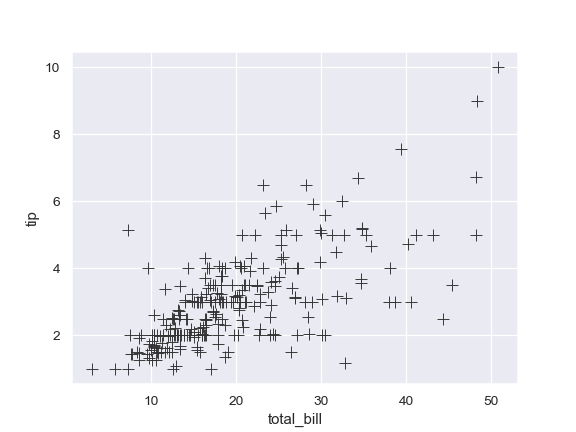Pass data vectors instead of names in a data frame:

```>>> iris = sns.load_dataset("iris")
>>> ax = sns.scatterplot(x=iris.sepal_length, y=iris.sepal_width,
...                      hue=iris.species, style=iris.species)
```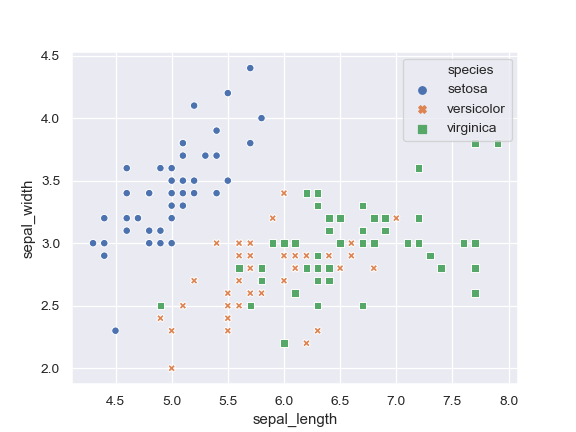Pass a wide-form dataset and plot against its index:

```>>> import numpy as np, pandas as pd; plt.close("all")
>>> index = pd.date_range("1 1 2000", periods=100,
...                       freq="m", name="date")
>>> data = np.random.randn(100, 4).cumsum(axis=0)
>>> wide_df = pd.DataFrame(data, index, ["a", "b", "c", "d"])
>>> ax = sns.scatterplot(data=wide_df)
```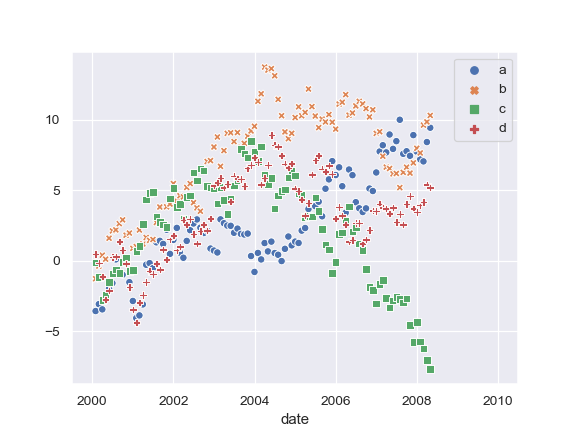Use `relplot()` to combine `scatterplot()` and `FacetGrid`: This allows grouping within additional categorical variables. Using `relplot()` is safer than using `FacetGrid` directly, as it ensures synchronization of the semantic mappings across facets.

```>>> g = sns.relplot(x="total_bill", y="tip",
...                  col="time", hue="day", style="day",
...                  kind="scatter", data=tips)
```